### Important TorchVision Random Transforms used in Image Augmentation - Python PyTorch

Wondered what Torch Vision transforms actually do? These transforms are most common in deep learning to perform image data augmentation. These are common image transformations used different application of computer vision and image processing.

In this post we will try to understand seven random transforms very important for image data augmentation. So what is a random transform. A random transformation will produce different result each time it is applied to an image.

#### What is Image Augmentation?

Through image augmentation we can create more image date by applying different transformations on the existing data. Applying these transformation we basically alter the original image so that we can create a bigger dataset. This dataset is used to train deep learning models.

#### Why random transforms are important for image augmentation?

The random transforms are more important to create the a bigger dataset with different types of images which are not available in the original dataset. So training process makes more robust.

#### What is importance of image data augmentation?

To create an image dataset is more time consuming and costly. So with sufficient images we can create a bigger dataset using image data augmentation.

## Import Required Libraries

`import torchimport torchvision.transforms as Tfrom PIL import Image`
The above imports are required to perform the transforms.
We  use PIL to read and display the image. You can use torchvision.io.read_image(path)  to read the image as a PyTorch tensor.

The next step to read the input image. We use the following image "Koala.jpg" to demonstrate all transformations.
`img = Image.open('Koala.jpg')img.show()`

Note: All transforms accepts both PIL image and Tensor. If the input image is a torch Tensor, it must be in [..., H, W] shape.

## RandomCrop

The RandomCrop transform crops the input image at random location.

#### Syntax

`torchvision.transforms.RandomCrop(size)`
Parameter: size- it is the expected size of cropped output image.

#### Example

`cropper = T.RandomCrop(size=(256, 256))cropped_img = cropper(img)cropped_img.show()`
Output: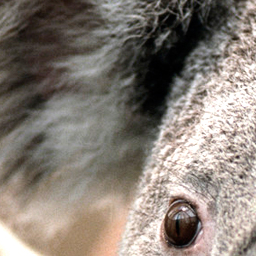Output- Random cropped image

Reference: torchvision.transforms.RandomCrop

## RandomResizedCrop

It crops a random portion of image and resize it to a given size. A crop of the original image is made and this crop is finally resized to the given size. It's used to train the Inception networks.

#### Syntax

`torchvision.transforms.RandomResizedCrop(size)`
Parameter: size- it is the expected size of cropped output image.

#### Example:

`resized_cropper = T.RandomResizedCrop(size=(256, 256))resized_crop = resized_cropper(img)resized_crop.show()`
Output: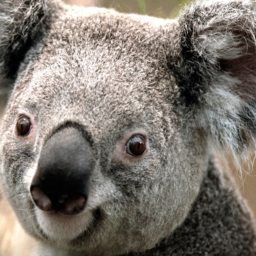Output: Random resize cropped image

## RandomRotation

It rotate the image by an angle.

#### Syntax

`torchvision.transforms.RandomRotation(degrees)`
Parameter: degrees - it is range of degree (min, max). The angle by which the image is rotated is selected from this range.

#### Example

`rotater = T.RandomRotation(degrees=(0, 180))rotated_img = rotater(img)rotated_img.show()`
Output: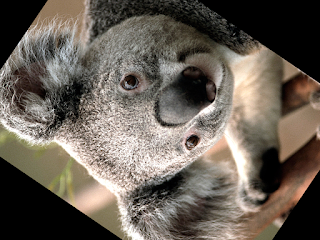Output: random rotated image

Reference: torchvision.transforms.RandomRotation

## RandomAffine

It make affine transformation of the image keeping center invariant.

#### Syntax

`torchvision.transforms.RandomAffine(degrees)`
Parameter: degrees -  it is range of degree (min, max). The angle by which the image is rotated is selected from this range.

#### Example:

`affine_transfomer = T.RandomAffine(degrees=(30, 70), translate=(0.1, 0.3), scale=(0.5, 0.75))affine_img = affine_transfomer(img)affine_img.show()`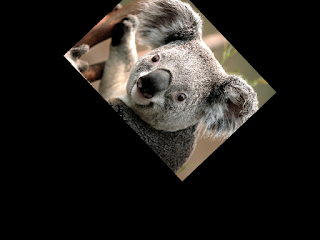Output: Random affined image

Reference: torchvision.transforms.RandomAffine

## GaussianBlur

It blurs image with randomly chosen Gaussian blur.

#### Syntax

`torchvision.transforms.GaussianBlur(kernel_size, sigma=(0.1, 2.0))`
Parameter: kernel_size- it is size of Gaussian kernel.

#### Example

`blurrer = T.GaussianBlur(kernel_size=(5, 9), sigma=(0.1, 5))blurred_img = blurrer(img)blurred_img.show()`
Output: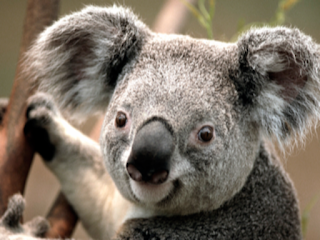Output: Gaussian Blur Image

## RandomInvert

It inverts the colors of the given image randomly with a given probability.

#### Syntax

`torchvision.transforms.RandomInvert(p=0.5)`

#### Example

```inverter = T.RandomInvert()invertered_img = inverter(img)
invertered_img.show()```
Output: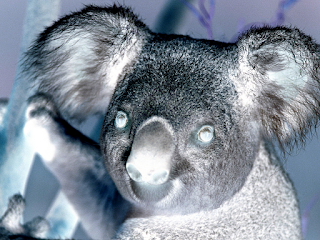Output: Random Inverted Image

## ColorJitter

It randomly change the brightness, contrast, saturation and hue of an image.

#### Syntax

`torchvision.transforms.ColorJitter(brightness=0, contrast=0, saturation=0, hue=0)`

#### Example:

`jitter = T.ColorJitter(brightness=.5, hue=.3)jitted_img = jitter(img)jitted_img.show()`
Output:Output: Random Color Jitter Image

We will discuss the following random transforms in the next tutorial-
• RandomPerspective
• RandomPosterize
• RandomSolarize
• RandomAutocontrast
• RandomEqualize
• AutoAugment
• RandAugment
Randomly Applied Transforms
• RandomHorizontalFlip
• RandomVerticalFlip
• RandomApply